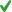Exemplary Writers

# Exchange-rate overshooting

The following graph shows the short-run supply schedule (S0S0) and demand schedule (D0D0) for the Mexican peso. S1S1 denotes the long-run supply schedule of pesos. The initial equilibrium exchange rate is $2.00 per peso. Suppose that the demand for pesos increases to D1D1. On the graph, use the tan point (dash symbol) to indicate the short-run equilibrium exchange rate. Then use the grey point (star symbol) to indicate the long-run equilibrium exchange rate. Note: Dashed drop lines will automatically extend to both axes. Points: 1 / 1 Close Explanation Explanation: Initially, the equilibrium exchange rate is at the intersection of the short-run supply schedule (S0S0) and demand schedule (D0D0) and is equal to$2.00 per peso. When the demand for pesos increases to D1D1, the dollar depreciates and the exchange rate changes to $3.50 per peso (the intersection of S0S0 and D1D1). In the long run, however, the supply of pesos will increase, and over time, the long-run supply curve will become flatter and more elastic than the short-run supply curve. So, in the long run, the equilibrium exchange rate will appreciate to$2.50 per peso (the intersection of S1S1 and D1D1).
In financial markets, such as foreign exchange markets, the price adjusts instantaneously, whereas in the goods markets, prices do not respond immediately to changes in market fundamentals. This creates an excessive movement in exchange rates during the short run, a phenomenon known as overshooting. As the general price level slowly gravitates to its new equilibrium level, the amount of the overshooting exchange rate dissipates, and the exchange rate moves toward its long-run equilibrium level.
Referring to the previous graph, use the dropdown menus in the following table to show the order of the events that led to the long-run equilibrium exchange rate.
Step
Event
1. The dollar depreciates to $3.50 per peso.2. The Mexican price of U.S. exports decreases, and the quantity of U.S. exports demanded increases.3. The quantity of pesos supplied increases.4. The supply schedule of pesos becomes more elastic, as shown by S1S1.5. The dollar appreciates to$2.50 per peso.Points:
1 / 1
Close Explanation
Explanation:
Step
Event
1. Immediately after the demand for pesos increases to D1D1, the dollar depreciates to $3.50 per peso. 2. Because of the dollar depreciation, the Mexican price of U.S. exports decreases, and the quantity of U.S. exports demanded increases. 3. As time passes, the greater the increase in the quantity of exports, the greater will be the increase in the quantity of pesos supplied. 4. The long-run supply curve becomes more elastic than the short-run supply curve, as shown by S1S1 and S0S0, respectively. 5. Following the increase in the demand for pesos to D1D1, the long-run equilibrium exchange rate appreciates to$2.50 per peso. This difference in elasticities of S0S0 and S1S1 explains why the dollar’s depreciation in the short run overshoots its depreciation in the long run.
Note that changes in the long-run value of the exchange rate are due to the reactions of traders in the foreign exchange market to changes in four key factors: relative price levels, relative productivity levels, consumer preferences for domestic or foreign goods, and trade barriers. These factors underlie trade in domestic and foreign goods and, thus, changes in the demand for exports and imports.